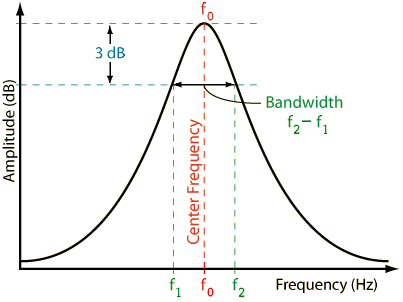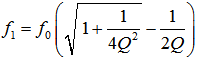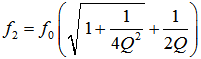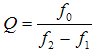Deutsche VersionCalculationEqualizationBandpassFilter

Calculating the bandwidth at −3 dB  cut-off frequencies  f1 and f2
when
center frequency  f0 and Q factor is given.
The bandwidth
BW is between lower and upper cut-off frequency.3 dB bandwidth BW = f2 f1= f0/Q and quality factor is Q factor
EQ filter conversion Q factor to bandwidth in octaves N
Parametric peak equalizer and notch (dip) equalizer
People use 'Q' and 'bandwidth' interchangeably, though they're not.
Defining the bandwidth for a bandpass as the −3 dB points cannot be correct for a boost gain of 3 dB or less.

f1 and f2 = corner frequency = cut-off frequency = crossover frequency
= half-power frequency = 3 dB frequency = break frequency is all the same.

The center frequency  f0 is the geometric mean of f1 and f2
BW = Δf = f0 / Q
Q = f0 / BW          f0 = BW × Q = √ (f1 × f2)
BW = f2 − f1            f1 = f02 / f2 = f2 BW             f2 = f02 / f1 = f1 + BW

 Center frequency f0 Hz Q factor or quality factor Q ↓ Lower cutoff frequency f1 Hz Upper cutoff frequency f2 Hz
 Formula for the lower cutoff frequency:Formula for the upper cutoff frequency:Formula for the Q factor:Formula for the bandwidth:A high filter quality means narrow-band filtering (notch), with a large Q factor.  This results in steep filter flanks with a small bandwidth.    A low filter quality means broad-band filtering, with a small Q factor.  This results in flat filter flanks with a large bandwidth.

 Notice:  A low Q factor gives a broad band (wide) bandwidth or  a high Q factor gives a narrow band (small) bandwidth.

Q factor as a function of the bandwidth in octaves N (octave bandwidth)

 Bandwidth in octaves N Q factor 3.0 wide 0.404 low 2.5 0.511 2.0 0.667 1.5 0.920 1.0 1.414 2/3 2.145 1/2 2.871 1/3 4.318 1/6 8.651 1/12 small 17.310 high

 Why is the bandwidth and the cutoff frequency found at the level of "−3 dB"? Why we always take 3 dB down gain of a filter? Full width at half maximum (FWHM). Answer: That is the point where the energy (power) is fallen to the value ½ or 0.5 = 50 percent of the initial power as energy quantity, that is equivalent to (−)3 dB = 10×log(0.5). A (−)3 dB power drop is a decrease of 50 % to the value of 50 %. There the voltage is fallen to the value of √(½) or 0.7071 = 70.71 percent of the initial voltage as field quantity equivalent to (−)3 dB = 20×log(0.7071). A (−)3 dB voltage drop is a decrease of 29.29 % to the value of 70.71 %.

 (−)3 dB implies ½ the electric power and since the power is proportional to the square of voltage, the value will be 0.7071 or 70.71 % of the passband voltage. √½ = 1/√2 = √0.5 = 0,7071. P ~ V2, that is 0,5 ~ 0,70712.

 Sound engineers and sound designers ("ear people") mostly use the usual (sound) field quantity. That'swhy they say: The cutoff frequency of a device (microphone, amplifier, loudspeaker) is the frequency at which the output voltage level is decreased to a value of (−)3 dB below the input voltage level (0 dB). ● (−)3 dB corresponds to a factor of √½ = 1/√2 = 0.7071, which is 70.71% of the input voltage.   Acousticians and sound protectors ("noise fighters") seem to like more the (sound) energy quantity. They tell us: The cutoff frequency of a device (microphone, amplifier, loudspeaker) is the frequency at which the output power level is decreased to a value of (−)3 dB below the input power level (0 dB). ● (−)3 dB corresponds to a factor of ½ = 0.5, which is 50% of the input power (half the value).

 Note: Power gain (power amplification) is not common in audio engineering. Even power amplifiers for loudspeakers don't amplify the power. They amplify the audio voltage that moves the voice coil.

 Sound field quantitiesSound pressure, sound or particle velocity, particle displacement or displacement amplitude, (voltage, current, electric resistance). Inverse Distance Law 1/r Sound energy quantities Sound intensity, sound energy density, sound energy, acoustic  power. (electrical power). Inverse Square Law 1/r²

 Note: A sound field quantity (sound pressure p, electric voltage V) is not a sound energy quantity (sound intensity I, sound power Pak). I ~ p2 or P ~ V2. Sometimes you can hear the statement: The cutoff frequency is there where the level L is decreased by (−)3 dB. Whatever the user wants to tell us so accurately: Level is level or dB is dB.

Bandwidth for Yamaha Parametric Equalizer

 For a Yamaha parametric equalizer EQ there is the filter bandwidth of an octave divided in 60/60 (12 semitones). One half tone step (semitone) is then 5/60 − 01V Digital Mixing Console. Conversion: N = "bandwidth in octaves" (semi tone or half tone distance). Q = Q factor

 Filter EQ N Q Interval Filter EQ N Q Interval 5/60 0.083 17.31 Semitone step 95/60 1.583 0.867 10/60 0.167 8.651 Whole tone 100/60 1.667 0.819 15/60 0.25 5.764 105/60 1.75 0.776 20/60 0.333 4.318 1/3 octave 110/60 1.833 0.736 25/60 0.417 3.45 115/60 1.917 0.7 30/60 0.5 2.871 1/2 octave 120/60 2 0.667 2 octaves 35/60 0.583 2.456 Fifth 125/60 2.083 0.636 40/60 0.667 2.145 130/60 2.167 0.607 45/60 0.75 1.902 135/60 2.25 0.581 50/60 0.833 1.707 140/60 2.333 0.556 55/60 0.917 1.548 145/60 2.417 0.532 60/60 1 1.,414 1 octave 150/60 2.5 0.511 2.5 octaves 65/60 1.083 1.301 155/60 2.583 0.49 70/60 1.67 1.204 160/60 2.667 0.471 75/60 1.25 1.119 165/60 2.,75 0.453 80/60 1.333 1.044 170/60 2.883 0.436 85/60 1.417 0.979 175/60 2.917 0.419 90/60 1.5 0.92 1.5 octaves 180/60 3 0.404 3 octaves

 The "BW/60" control replicates the effect of the Behringer Pro DSP1124P - Feedback Destroyer bandwidth setting. This control sets the bandwidth of the filter between the half-gain points with: BW (Hz) = f0 × (BW / 60) × √2 For example, at a bandwidth setting of 60/60 a filter centred on 1 kHz with a gain of −6 dB will have a bandwidth of 1,414 Hz between the points where its response crosses −3 dB. This bandwidth remains constant as the filter's gain is adjusted. Note that the Behringer DSP1100 - 24 band parametric equalizer software package does NOT correctly reproduce the way the bandwidth control actually operates, its bandwidths are too small by a factor of √2. Defining filter bandwidth in this way is not uncommon (the TMREQ filters use a similar definition). The relationship between Q and BW for the DSP1124P is: Q = 60 / [(BW / 60) × √2] So the bandwidth range of 1/60 to 120/60 gives a range from Q = 42.4 to 0.35.At the cut-off frequency fc of a drop the voltage V is always fallen to the value 1/√2 = 0.7071 ≡ 70.71 % and the voltage level is damped by 20 × log (1/√2) = (−)3.0103 dB. At the cut-off frequency (half-power frequency) the less interesting power P is always fallen to 1/2 = 0.5 ≡ 50 % and the power level is damped by 10 × log (1/2) = (−)3.0103 dB − that is the same dB value. This is often confusing. 0.7071 × 0.7071 is 0.5 and P = V²/R; P ~ V². What do you mean by 3 dB cutoff frequency? Why is it 3 dB, not 1 dB? Answer: The power P is always fallen there to 1/2 = 0.5 = 50 %.

Quality Factor Q = f0 / BW
BW = f0/Q          Q = f0/BW          f0= BW × Q

Please enter two values, the third value will be calculated.

 Center frequency f0 HzBandwidth BW Hz Quality Factor Q
Measurement of input impedance and output impedance